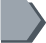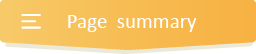Your browser does not support JavaScript!

#Grade 6 adding and subtracting fractions activities with answers### How to add and subtract fractions with like denominators.

The easiest way to learn how to add and subtract fractions with like denominators is to think of addition and subtraction of whole numbers. Here, since the denominators are the same, add or subtract the numerators, then write the result over the same denominator.

### How to add and subtract fractions with unlike denominators

To successfully add and subtract fractions with unlike denominators, you need to follow the steps below:

•  Find the least common multiple (LCM) of the denominators. To find the LCM of the denominators, find the multiples of each denominator. Then, look for the lowest multiple that is common to both denominators. That will be the LCM.

•  Rewrite each fraction to its equivalent fraction with a denominator equal to the least common multiple(LCM).

• Add or subtract the numerator as required in the problem. The answer's denominator will be the same as the denominator of the fractions being added or subtracted.

•